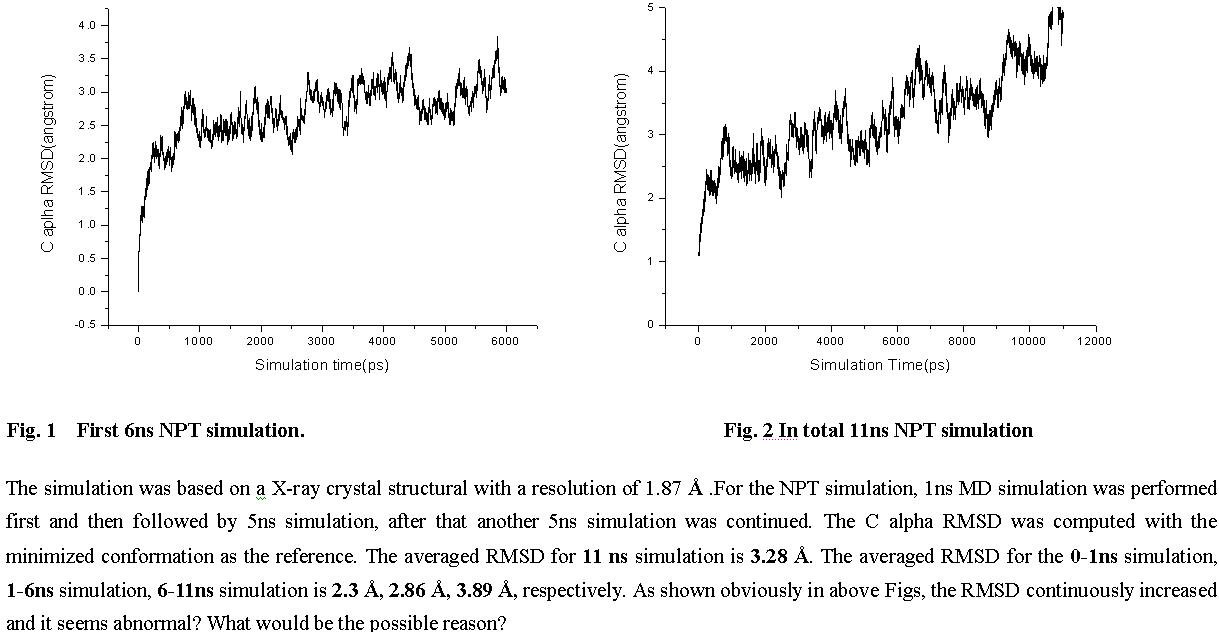# [AMBER] Why RMSD goes fast to 5 angstrom?

From: Shulin Zhuang <shulin.zhuang.gmail.com>
Date: Wed, 18 Apr 2012 10:26:20 +0800

Dear All,

I have routinely performed a 11 ns MD simulation in NPT ensemble based on
X-ray crystal structure with a resolution of 1.87 angstrom . After the RMSD
analysis, I found that the C alpha RMSD is continiously increasing and
finally is is up to *5 Ĺ*. The averaged RMSD for the* 0-1ns* simulation, *
1-6ns* simulation, *6-11ns* simulation is *2.3** Ĺ, 2.86 Ĺ, 3.89
Ĺ,*respectively. Attached
is the RMSD figures.* It seems abnormal* and could you tell me where is the
problem.

The simulatioin input files were listed as following:
*
Minimization step 1 input:*

restrained mimimization

&cntrl

imin=1, maxcyc=1000, ncyc=500, cut=10.0, ntb=1,

ntr=1, restraintmask='(:268) & (!.H=)', restraint_wt=10.0

# here 268 is the ligand. In this step, the ligand and non-hydrogen part of
the system were restrained.

/

*Minimization step 2 input:*

restrained mimimization

&cntrl

imin=1,maxcyc=1000, ncyc=500, cut=10.0, ntb=1, ntr=0,

/

*Heating stage input:*

restrained heating process

&cntrl

imin=0, irest = 0, ntx = 1, ntb = 1, ntr = 1, ntc= 2, tempi = 0.0,
temp0 = 300.0,

ntt = 3, gamma_ln = 1.0, nstlim = 25000, dt = 0.002, ntpr = 100, ntwx
= 500, ntwr = 500,

cut=10.0, restraintmask='(:268) & (!.H=)', restraint_wt=5.0,

******

*/*

*
*

*1ns equilibration input:*

&cntrl

ntx =7, ntr = 0, irest = 1, imin = 0, nrespa = 1, ntb =2, ntp=1,

tempi =300.0, temp0 =300.0,cut=10.0, nstlim =500000, dt= 0.002, ntpr=200,

ntc=2, ntf=2, taup = 2, pres0 = 1.0, ntwr=500, ntwx=500, ntt=3,
gamma_ln=1.0,

*5ns equilibration input:*

&cntrl

ntx =5, ntr = 0, irest = 1, imin = 0, nrespa = 1, ntb =2, ntp=1, tempi
=300.0,

temp0 =300.0,cut=10.0, nstlim =2500000, dt= 0.002, ntpr=200, ntc=2,
ntf=2, taup = 2, pres0 = 1.0,

ntwr=500, ntwx=500, ntt=3, gamma_ln=1.0,

*5ns equilibration input:*
&cntrl
ntx =5, ntr = 0, irest = 1, imin = 0, nrespa = 1, ntb =2, ntp=1,
tempi =300.0, temp0 =300.0,cut=10.0, nstlim =2500000, dt= 0.002,
ntpr=200,
ntc=2, ntf=2, taup = 2, pres0 = 1.0, ntwr=500, ntwx=500, ntt=3,
gamma_ln=1.0,
/

Much appreciated to your help!

Shulin

_______________________________________________
AMBER mailing list
AMBER.ambermd.org
http://lists.ambermd.org/mailman/listinfo/amber(image/jpeg attachment: RMSD-figure.jpg)

Received on Tue Apr 17 2012 - 19:30:02 PDT
Custom Search